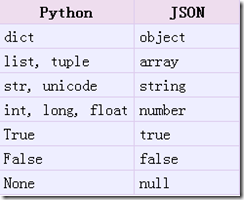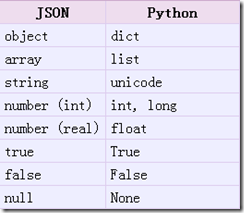# Json概述以及python对json的相关操作

JSON(JavaScript Object Notation) 是一种轻量级的数据交换格式。易于人阅读和编写。同时也易于机器解析和生成。它基于JavaScript Programming Language, Standard ECMA-262 3rd Edition - December 1999的一个子集。JSON采用完全独立于语言的文本格式，但是也使用了类似于C语言家族的习惯（包括C, C++, C#, Java, JavaScript, Perl, Python等）。这些特性使JSON成为理想的数据交换语言。

JSON建构于两种结构：

“名称/值”对的集合（A collection of name/value pairs）。不同的语言中，它被理解为对象（object），纪录（record），结构（struct），字典（dictionary），哈希表（hash table），有键列表（keyed list），或者关联数组 （associative array）。

jso官方说明参见：http://json.org/

Python操作json的标准api库参考：http://docs.python.org/library/json.html

import json

obj = [[1,2,3],123,123.123,'abc',{'key1':(1,2,3),'key2':(4,5,6)}]
encodedjson = json.dumps(obj)
print repr(obj)
print encodedjson

[[1, 2, 3], 123, 123.123, 'abc', {'key2': (4, 5, 6), 'key1': (1, 2, 3)}]
[[1, 2, 3], 123, 123.123, "abc", {"key2": [4, 5, 6], "key1": [1, 2, 3]}]decodejson = json.loads(encodedjson)
print type(decodejson)
print decodejson['key1']
print decodejson

<type 'list'>
[1, 2, 3]

[[1, 2, 3], 123, 123.123, u'abc', {u'key2': [4, 5, 6], u'key1': [1, 2, 3]}]json.dumps方法提供了很多好用的参数可供选择，比较常用的有sort_keys（对dict对象进行排序，我们知道默认dict是无序存放的），separators，indent等参数。

data1 = {'b':789,'c':456,'a':123}
data2 = {'a':123,'b':789,'c':456}
d1 = json.dumps(data1,sort_keys=True)
d2 = json.dumps(data2)
d3 = json.dumps(data2,sort_keys=True)
print d1
print d2
print d3
print d1==d2
print d1==d3

{"a": 123, "b": 789, "c": 456}
{"a": 123, "c": 456, "b": 789}
{"a": 123, "b": 789, "c": 456}
False
True

indent参数是缩进的意思，它可以使得数据存储的格式变得更加优雅。

data1 = {'b':789,'c':456,'a':123}
d1 = json.dumps(data1,sort_keys=True,indent=4)
print d1

{
"a": 123,
"b": 789,
"c": 456
}

print 'DATA:', repr(data)
print 'repr(data)             :', len(repr(data))
print 'dumps(data)            :', len(json.dumps(data))
print 'dumps(data, indent=2)  :', len(json.dumps(data, indent=4))
print 'dumps(data, separators):', len(json.dumps(data, separators=(',',':')))

DATA: {'a': 123, 'c': 456, 'b': 789}
repr(data)             : 30
dumps(data)            : 30
dumps(data, indent=2)  : 46
dumps(data, separators): 25

data = {'b':789,'c':456,(1,2):123}
print json.dumps(data,skipkeys=True)

{"c": 456, "b": 789}

json模块不仅可以处理普通的python内置类型，也可以处理我们自定义的数据类型，而往往处理自定义的对象是很常用的。

class Person(object):
def __init__(self,name,age):
self.name = name
self.age = age
def __repr__(self):
return 'Person Object name : %s , age : %d' % (self.name,self.age)
if __name__  == '__main__':
p = Person('Peter',22)
print p

'''
Created on 2011-12-14
@author: Peter
'''
import Person
import json

p = Person.Person('Peter',22)

def object2dict(obj):
#convert object to a dict
d = {}
d['__class__'] = obj.__class__.__name__
d['__module__'] = obj.__module__
d.update(obj.__dict__)
return d

def dict2object(d):
#convert dict to object
if'__class__' in d:
class_name = d.pop('__class__')
module_name = d.pop('__module__')
module = __import__(module_name)
class_ = getattr(module,class_name)
args = dict((key.encode('ascii'), value) for key, value in d.items()) #get args
inst = class_(**args) #create new instance
else:
inst = d
return inst

d = object2dict(p)
print d
#{'age': 22, '__module__': 'Person', '__class__': 'Person', 'name': 'Peter'}

o = dict2object(d)
print type(o),o
#<class 'Person.Person'> Person Object name : Peter , age : 22

dump = json.dumps(p,default=object2dict)
print dump
#{"age": 22, "__module__": "Person", "__class__": "Person", "name": "Peter"}

#Person Object name : Peter , age : 22

JSONEncoder类负责编码，主要是通过其default函数进行转化，我们可以override该方法。同理对于JSONDecoder。

'''
Created on 2011-12-14
@author: Peter
'''
import Person
import json

p = Person.Person('Peter',22)

class MyEncoder(json.JSONEncoder):
def default(self,obj):
#convert object to a dict
d = {}
d['__class__'] = obj.__class__.__name__
d['__module__'] = obj.__module__
d.update(obj.__dict__)
return d

class MyDecoder(json.JSONDecoder):
def __init__(self):
json.JSONDecoder.__init__(self,object_hook=self.dict2object)
def dict2object(self,d):
#convert dict to object
if'__class__' in d:
class_name = d.pop('__class__')
module_name = d.pop('__module__')
module = __import__(module_name)
class_ = getattr(module,class_name)
args = dict((key.encode('ascii'), value) for key, value in d.items()) #get args
inst = class_(**args) #create new instance
else:
inst = d
return inst

d = MyEncoder().encode(p)
o =  MyDecoder().decode(d)

print d
print type(o), o

posted @ 2011-12-14 16:21 糖拌咸鱼 阅读(...) 评论(...) 编辑 收藏